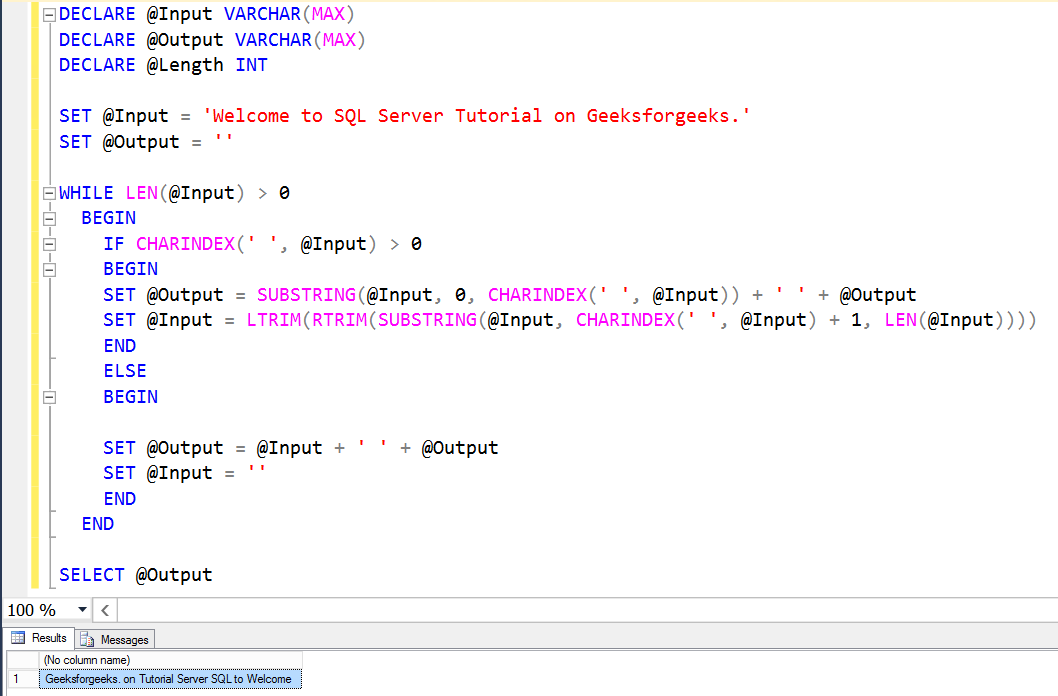# Reverse Statement Word by Word in SQL server

• Last Updated : 01 Oct, 2020

To reverse any statement Word by Word in SQL server we could use the SUBSTRING function which allows us to extract and display the part of a string.

Pre-requisite :SUBSTRING function

Approach :

• Declared three variables (@Input, @Output, @Length) using the DECLARE statement.
• Use the WHILE Loop to iterate every character present in the @Input. For the condition inside the SQL Server while loop, use the SQL LEN Function to check whether the length of the Input is greater than 0 or not.
• Within the while loop, use the SUBSTRING Function to set the @Output value with three parameters, and they are Input, start point, endpoint. Here we assigned the Input as @Input, use the start point as 0.
• Use the CHARINDEX Function to find the ‘ ‘, so that the endpoint will be before the space.
• Now, adding this to @Output, and which is empty at the starting of the loop.
• To update the @Input variable, use the SUBSTRING Function. Here we assigned the Input as @Input.
• Next, use the SQL CHARINDEX Function to find the empty space, and then we added 1 so that the starting point will be after space.
• Then, use the SQL LEN Function to specify the end value.
• At SET @Output it is extracting the word starting at 0 indexes and up to empty space. That will be the first word.
• Once it got its first word, it will remove that word from @Input using the SET @Input code.

Input :
Welcome to SQL Server Tutorial on Geeksforgeeks.

Example –

```DECLARE @Input VARCHAR(MAX)
DECLARE @Output VARCHAR(MAX)
DECLARE @Length INT

SET @Input = 'Welcome to SQL Server
Tutorial on Geeksforgeeks.'

SET @Output = ''
WHILE LEN(@Input) > 0

BEGIN
IF CHARINDEX(' ', @Input) > 0

BEGIN
SET @Output = SUBSTRING
(@Input, 0, CHARINDEX(' ', @Input))
+ ' ' + @Output
SET @Input = LTRIM
(RTRIM
(SUBSTRING
(@Input, CHARINDEX
(' ', @Input) + 1, LEN(@Input))))
END

ELSE

BEGIN
SET @Output = @Input + ' ' + @Output
SET @Input = ''
END
END
SELECT @Output
```

Output :

```Geeksforgeeks. on Tutorial Server SQL to Welcome
```

Input and Output using SQL Server Management Studio :My Personal Notes arrow_drop_up